# Basic Arithmetic : Whole Numbers

## Example Questions

1 2 3 5 Next →

### Example Question #1 : Solving Equations With Whole Numbers

Solve for t.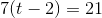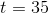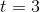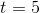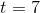Explanation:

First start by distributing the 7.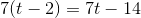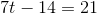Now, add both sides by 14.Finally, divide both sides by 7.### Example Question #1 : Solving Equations With Whole Numbers

Solve for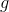.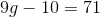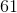Explanation:

Start by adding 10 to both sides of the equation.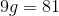Then, divide both sides by.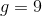### Example Question #1 : Solving Equations With Whole Numbers

Solve for: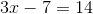Explanation:

First, addto both sides of the equation: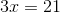Then, divide both sides by: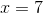### Example Question #2 : Solving Equations With Whole Numbers

If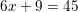, what isequal to?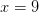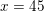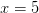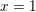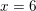Explanation:

When solving an equation, we need to find a value of x which makes each side equal each other. We need to remember that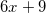is equal to and the same as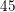. When we solve an equation, if we make a change on one side, we therefore need to make the exact same change on the other side, so that the equation stays equal and true. To illustrate, let's take a numerical equation: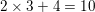If we subtract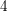from each side, the equation still remains equal: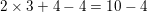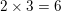If we now divide each side by, the equation still remains equal: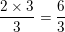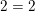This still holds true even if we have variables in our equation. We can perform the inverse operations to isolate the variable on one side and find out what number it's equal to. To solve our problem then, we need to isolate ourterm. We can do that by subtractingfrom each side, the inverse operation of adding: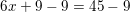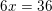We now want there to be oneon the left side.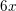is the same thing as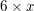, so we can get rid of the 6 by performing the inverse operation on both sides, i.e. dividing each side by: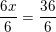is therefore our final answer.

### Example Question #2 : Solving Equations With Whole Numbers

Solve: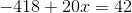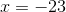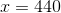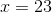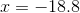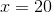Explanation:

The answer is. The goal is to isolate the variable,, on one side of the equation sign and have all numerical values on the other side of the equation.

Since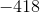is a negative number, you must add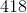to both sides.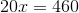Then, divide both sides of the equation by: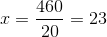1 2 3 5 Next →

### All Basic Arithmetic Resources